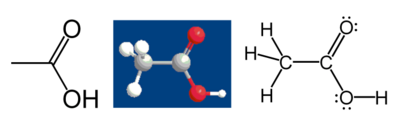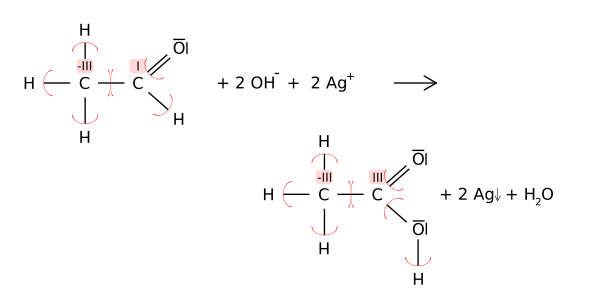# Oxidation state

In chemistry, the oxidation state is an indicator of the degree of oxidation of an atom in a chemical compound. The formal oxidation state is the hypothetical charge that an atom would have if all bonds to atoms of different elements were 100 percent ionic. Oxidation states are represented by Arabic numerals and can be positive, negative, or zero.

The increase in oxidation state of an atom is known as an oxidation; a decrease in oxidation state is known as a reduction. Such reactions involve the formal transfer of electrons, a net gain in electrons being a reduction and a net loss of electrons being an oxidation. In this manner, the concept of oxidation state helps scientists understand oxidation-reduction (redox) chemical reactions.

## History

The concept of oxidation state in its current meaning was introduced by W. M. Latimer in 1938. Oxidation itself was first studied by Antoine Lavoisier, who held the belief that oxidation was literally the results of reactions of the elements with oxygen and that the common bond in any salt was based on oxygen.

## Official definition and rules

Here is the definition of the oxidation state, according to the International Union of Pure and Applied Chemistry (IUPAC):

Oxidation state: A measure of the degree of oxidation of an atom in a substance. It is defined as the charge an atom might be imagined to have when electrons are counted according to an agreed-upon set of rules: (l) the oxidation state of a free element (uncombined element) is zero; (2) for a simple (monatomic) ion, the oxidation state is equal to the net charge on the ion; (3) hydrogen has an oxidation state of 1 and oxygen has an oxidation state of -2 when they are present in most compounds. (Exceptions to this are that hydrogen has an oxidation state of -1 in hydrides of active metals, e.g. LiH, and oxygen has an oxidation state of -1 in peroxides, e.g. H2O2; (4) the algebraic sum of oxidation states of all atoms in a neutral molecule must be zero, while in ions the algebraic sum of the oxidation states of the constituent atoms must be equal to the charge on the ion. For example, the oxidation states of sulfur in H2S, S8 (elementary sulfur), SO2, SO3, and H2SO4 are, respectively: -2, 0, +4, +6 and +6. The higher the oxidation state of a given atom, the greater is its degree of oxidation; the lower the oxidation state, the greater is its degree of reduction.

## Calculation of formal oxidation states

There are two common ways of computing the oxidation state of an atom in a compound. The first one is used for molecules when one has a Lewis structure, as is often the case for organic molecules, while the second one is used for simple compounds (molecular or not) and does not require a Lewis structure.

It should be remembered that the oxidation state of an atom does not represent the "real" charge on that atom: this is particularly true of high oxidation states, where the ionization energy required to produce a multiply positive ion are far greater than the energies available in chemical reactions. The assignment of electrons between atoms in calculating an oxidation state is purely a formalism, albeit a useful one for the understanding of many chemical reactions.

For more about issues with calculating atomic charges, see partial charge.

### From a Lewis structure

When a Lewis structure of a molecule is available, the oxidation states may be assigned unambiguously by computing the difference between the number of valence electrons that a neutral atom of that element would have and the number of electrons that "belong" to it in the Lewis structure. For purposes of computing oxidation states, electrons in a bond between atoms of different elements belong to the most electronegative atom; electrons in a bond between atoms of the same element are split equally, and electrons in lone pair belong only to the atom with the lone pair.

For example, consider acetic acid:The methyl group carbon atom has 6 valence electrons from its bonds to the hydrogen atoms because carbon is more electronegative than hydrogen. Also, 1 electron is gained from its bond with the other carbon atom because the electron pair in the C–C bond is split equally, giving a total of 7 electrons. A neutral carbon atom would have 4 valence electrons, because carbon is in group 14 of the periodic table. The difference, 4 – 7 = –3, is the oxidation state of that carbon atom. That is, if it is assumed that all the bonds were 100 percent ionic (which in fact they are not), the carbon would be described as C3-.

Following the same rules, the carboxylic acid carbon atom has an oxidation state of +3 (it only gets one valence electron from the C–C bond; the oxygen atoms get all the other electrons because oxygen is more electronegative than carbon). The oxygen atoms both have an oxidation state of –2; they get 8 electrons each (4 from the lone pairs and 4 from the bonds), while a neutral oxygen atom would have 6. The hydrogen atoms all have oxidation state +1, because they surrender their electron to the more electronegative atoms to which they are bonded.

Oxidation states can be useful for balancing chemical equations for redox reactions, because the changes in the oxidized atoms have to be balanced by the changes in the reduced atoms. For example, in the reaction of acetaldehyde with the Tollens' reagent to acetic acid (shown below), the carbonyl carbon atoms changes its oxidation state from +1 to +3 (oxidation). This oxidation is balanced by reducing two equivalents of silver from Ag+ to Ago.### Without a Lewis structure

The algebraic sum of oxidation states of all atoms in a neutral molecule must be zero, while in ions the algebraic sum of the oxidation states of the constituent atoms must be equal to the charge on the ion. This fact, combined with the fact that some elements almost always have certain oxidation states, allows one to compute the oxidation states for atoms in simple compounds. Some typical rules that are used for assigning oxidation states of simple compounds follow:

• Fluorine has an oxidation state of −1 in all its compounds, since it has the highest electronegativity of all reactive elements.
• Hydrogen has an oxidation state of +1 except when bonded to more electropositive elements such as sodium, aluminium, and boron, as in NaH, NaBH4, LiAlH4, where each H has an oxidation state of -1.
• Oxygen has an oxidation state of −2 except where it is −1 in peroxides, −1/2 in superoxides, −1/3 in ozonides, and of +2 in oxygen difluoride, OF2,+1 in O2F2.
• Alkali metals have an oxidation state of +1 in virtually all of their compounds (exception, see alkalide).
• Alkaline earth metals have an oxidation state of +2 in virtually all of their compounds.
• Halogens, other than fluorine have an oxidation state of −1 except when they are bonded to oxygen, nitrogen or with another halogen.

Example: In Cr(OH)3, oxygen has an oxidation state of −2 (no fluorine, O-O bonds present), and hydrogen has a state of +1 (bonded to oxygen). So, the triple hydroxide group has a charge of 3 × (−2 + 1) = −3. As the compound is neutral, Cr has an oxidation state of +3.

## Elements with multiple oxidation states

Most elements have more than one possible oxidation state, with carbon having nine, as follows:

1. –4: CH4
2. –3: C2H6
3. –2: CH3F
4. –1: C2H2
5.  0: CH2F2
6. +1: C2H2F4
7. +2: CHF3
8. +3: C2F6
9. +4: CF4

Oxygen has eight different oxidation states:

1. -2 in most oxides. For example, ZnO, CO2, H2O
2. -1 in all peroxides.
3. -1/2 as in superoxides. For example, KO2
4. -1/3 as in ozonides. For example, RbO3
5. 0 as in O2
6. +1/2 as in dioxygenyl. For example, O2+[AsF6]-
7. +1 in O2F2
8. +2 in OF2

### Fractional oxidation states

The formal oxidation state of an atom in a Lewis structure is always an integer. However, fractional oxidation states are often used to represent the average oxidation states of several atoms in a structure. For example, in KO2, oxygen has an average oxidation state of −½, which results from having one oxygen atom with oxidation state 0 and one with oxidation state −1. In some cases, the atoms may indeed be equivalent due to resonance; in those cases, the structure cannot be represented by a single Lewis structure—several structures are required.

## Oxidation number

The terms oxidation state and oxidation number are often used interchangeably. Rigorously, however, oxidation number is used in coordination chemistry with a slightly different meaning. In coordination chemistry, the rules used for counting electrons are different: Every electron belongs to the ligand, regardless of electronegativity. Also, oxidation numbers are conventionally represented with Roman numerals while oxidation states use Arabic numerals.